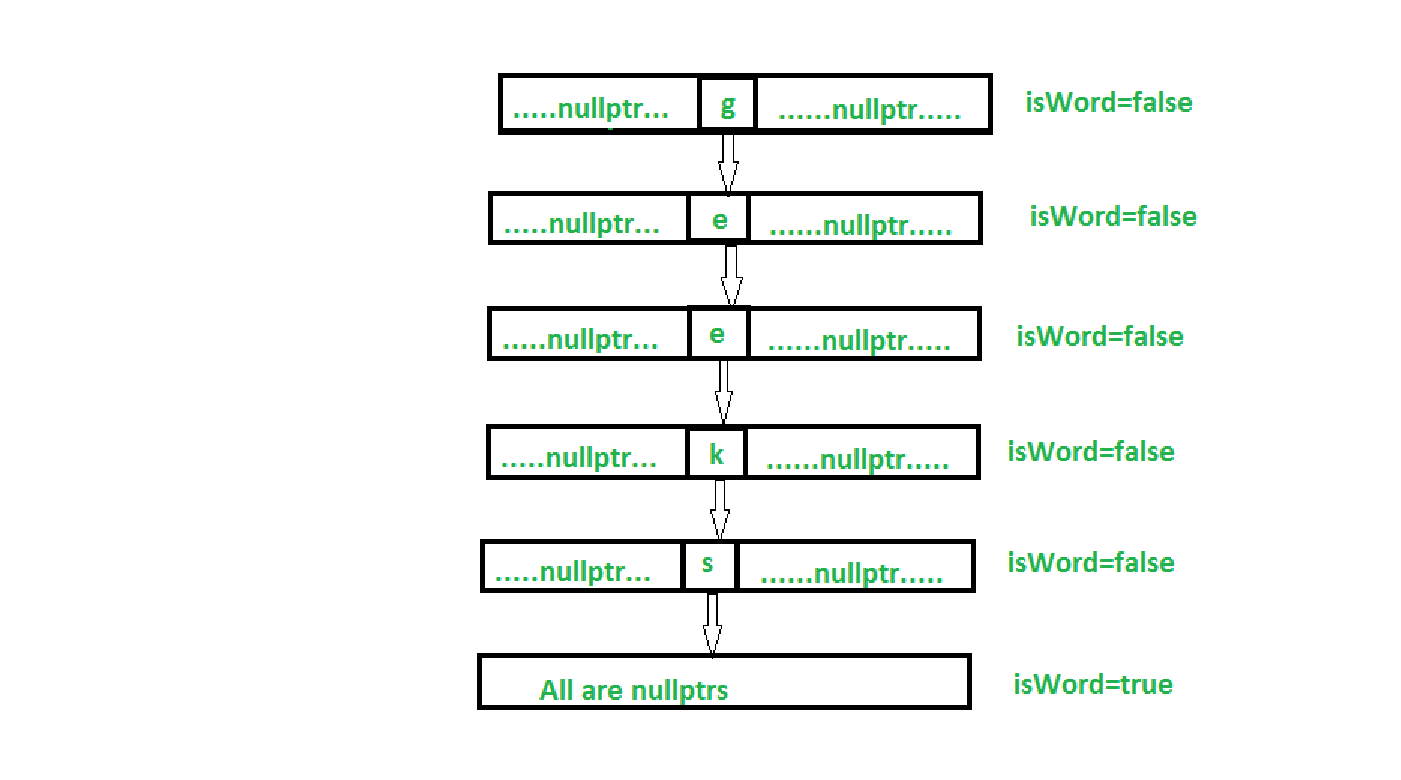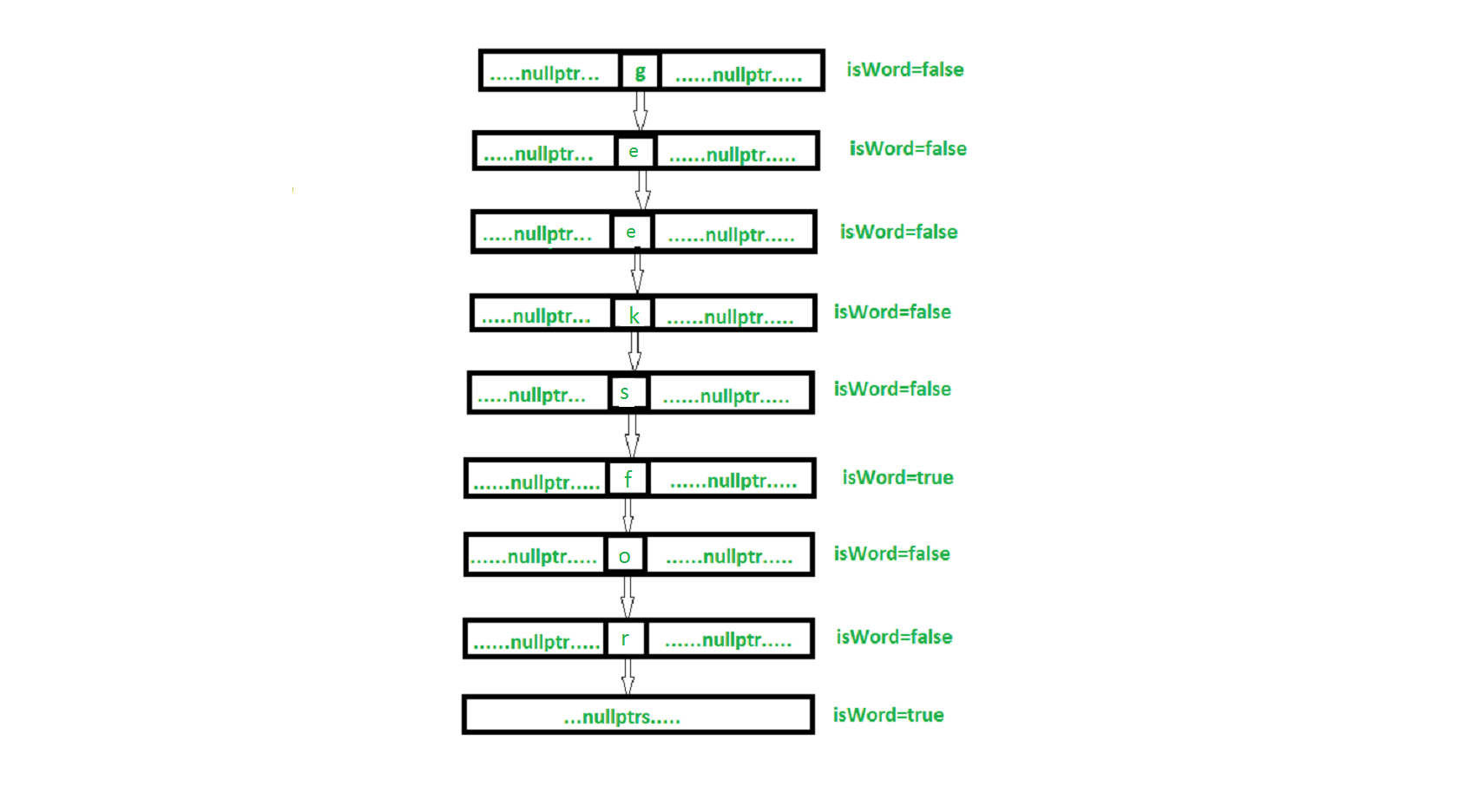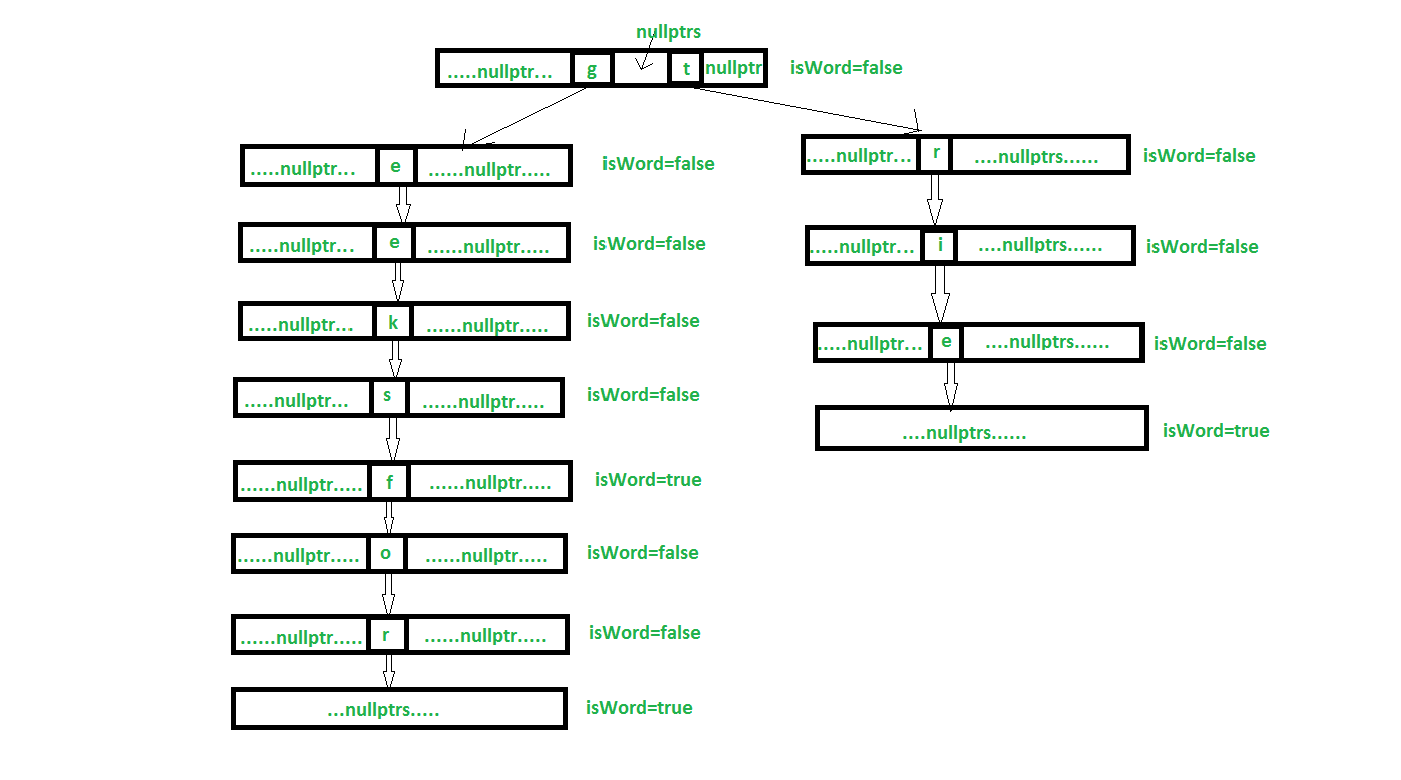# Trie Data Structure using smart pointer and OOP in C++

We will implement trie using smart pointers in C++ and OOP. Here, We have already discussed the implementation of trie data using recursion

In our implementation node of a trie look like :

 `class` `TrieNode{ ` ` `  `    ``public``: ` `    ``// Use of shared_ptr for storing Children  ` `    ``// Pointers of TrieNode ` ` `  `    ``shared_ptr children[ALPHABET_SIZE]; ` ` `  `    ``// Tracks whether If this Node is the end of  ` `    ``// any word stored in Trie ` ` `  `    ``bool` `isWord; ` ` `  `    ``// Constructor for TrieNode ` `    ``TrieNode() ` `    ``{ ` `        ``for``(``int` `i=0; i

The interface is the class showing the functionalities of our object class which has only pure virtual functions. Here, the concept interface discussed in more detail.

 `// Interface representing functionalities  ` `// of Trie Data Structure ` `class` `interface { ` `public``: ` `    ``interface() {} ` `    ``virtual` `~interface() {} ` `    ``// Pure virtual functions showing all  ` `    ``// the functions of my Trie class ` `  `  `    ``// To convert character to integer like hashing ` `    ``virtual` `int` `i2c(``char``) = 0; ` `  `  `    ``// Inserts word to Trie ` `    ``virtual` `void` `insertWord(string&) = 0; ` `  `  `    ``// Deletes word if present ` `    ``virtual` `bool` `deleteWord(string&) = 0; ` `  `  `    ``// To search a word in Trie ` `    ``virtual` `bool` `searchWord(string&) = 0; ` `  `  `    ``// Checks whether there is any children present ` `    ``// for any node ` `    ``virtual` `bool` `isEmptyNode(shared_ptr)  ` `                                          ``const` `= 0; ` `}; `

nullptrs stores NULL which suggests that there is no path for any other string from that position

Below image shows the insertion of “geeks” into an empty trie.Below image shows the insertion of “geeksfor” into the trie. geeks p is already exiting in the trie. so, it overlaps.Below image shows the insertion of “trie” into the trieWhy shared_ptr instead of all other smart pointers (auto_ptr, unique_ptr, weak_ptr)?

We are using shared_ptr due to the fact that while constructing the Trie we may encounter a situation where we may have to share the resource of a particular pointer to the other temporary shared_ptr to traverse Trie. In that shared_ptr is better than any other above mentioned pointers.

Below is the implementation of the above approach :

 `#include ` ` `  `// For shared_ptr class ` `#include   ` ` `  `using` `namespace` `std; ` `#define ALPHABET_SIZE 26 ` ` `  `// Trie Node class ` `class` `TrieNode { ` ` `  `public``: ` `    ``// Use of shared_ptr for storing Children ` `    ``// Pointers of TrieNode ` ` `  `    ``shared_ptr children[ALPHABET_SIZE]; ` ` `  `    ``// Tracks whether If this Node is the end of ` `    ``// any word stored in Trie ` ` `  `    ``bool` `isWord; ` ` `  `    ``// Constructor for TrieNode ` `    ``TrieNode() ` `    ``{ ` `        ``for` `(``int` `i = 0; i < ALPHABET_SIZE; i++) { ` `            ``children[i] = shared_ptr(); ` `        ``} ` `        ``isWord = ``false``; ` `    ``} ` `}; ` ` `  `// Interface representing functionalities  ` `// of Trie Data Structure ` `class` `interface { ` `public``: ` `    ``interface() {} ` `    ``virtual` `~interface() {} ` `    ``// Pure virtual functions showing all  ` `    ``// the functions of my Trie class ` ` `  `    ``// To convert character to integer like hashing ` `    ``virtual` `int` `i2c(``char``) = 0; ` ` `  `    ``// Inserts word to Trie ` `    ``virtual` `void` `insertWord(string&) = 0; ` ` `  `    ``// Deletes word if present ` `    ``virtual` `bool` `deleteWord(string&) = 0; ` ` `  `    ``// To search a word in Trie ` `    ``virtual` `bool` `searchWord(string&) = 0; ` ` `  `    ``// Checks whether there is any children present ` `    ``// for any node ` `    ``virtual` `bool` `isEmptyNode(shared_ptr)  ` `                                          ``const` `= 0; ` `}; ` ` `  `class` `Trie : ``public` `interface { ` `     `  `    ``// Storing root of the Trie ` `    ``shared_ptr root; ` `    ``int` `Total_words; ` ` `  `public``: ` `    ``Trie() ` `    ``{ ` `        ``root = shared_ptr ` `                              ``(``new` `TrieNode()); ` `        ``Total_words = 0; ` `    ``} ` ` `  `    ``~Trie() ` `    ``{ ` `        ``// Need not delete anything  ` `                ``// as shared_ptr deallocates  ` `                ``// all memory automatically ` `    ``} ` ` `  `    ``// To convert characters of string into ` `    ``// integer like hashing of character ` `    ``int` `i2c(``char` `c) ` `    ``{ ` `        ``return` `static_cast``<``int``>(c - ``'a'``); ` `    ``} ` ` `  `    ``// Insert word function ` `    ``void` `insertWord(string& trieString) ` `    ``{ ` `        ``shared_ptr current = root; ` `        ``for` `(``int` `i = 0; i < trieString.size(); i++)  ` `                ``{ ` `             `  `            ``// If word after some prefix is not  ` `                        ``// present then creates new node ` `            ``if` `(current->children[i2c(trieString[i])] ==  ` `                          ``shared_ptr(nullptr))  ` `            ``{ ` `                ``current->children[i2c(trieString[i])] =  ` `                     ``shared_ptr(``new` `TrieNode()); ` `            ``} ` `            ``current = (current->children[i2c(trieString[i])]); ` `        ``} ` ` `  `        ``// Now word is added in Trie so at leaf node  ` `        ``// for that word isWord=true ` `        ``current->isWord = ``true``; ` `    ``} ` ` `  `    ``// Searching for word whether it is  ` `    ``// present in Trie ` `    ``bool` `searchWord(string& trieString) ` `    ``{ ` `        ``shared_ptr current = root; ` ` `  `        ``for` `(``int` `i = 0; i < trieString.size(); i++)  ` `        ``{ ` `            ``// If at any point in Trie Node for particular  ` `            ``// character is not present means nullptr then  ` `            ``// return false ` `            ``if` `(current->children[i2c(trieString[i])] ==  ` `                            ``shared_ptr(nullptr)) ` `                ``return` `false``; ` `            ``current = current->children[i2c(trieString[i])]; ` `        ``} ` ` `  `        ``// At the end of the word checking whether this ` `        ``// word is really present or not ` `        ``if` `(current->isWord == ``true``) ` `            ``return` `true``; ` ` `  `        ``return` `false``; ` `    ``} ` ` `  `    ``bool` `Partdelete(string& trieString, shared_ptr&       ` `                                                     ``checkout) ` `    ``{ ` `        ``// Word is not present in the Trie then returns  ` `        ``// false and stops further recursion ` `        ``if` `(checkout == nullptr) ` `            ``return` `false``; ` ` `  `        ``// At the end of the word if the word is  ` `        ``// present in trie then setting isWord to  ` `        ``// false either returing false ` `        ``if` `(trieString.size() == 0) { ` `            ``if` `(checkout->isWord == ``true``) { ` `                ``checkout->isWord = ``false``; ` `                ``return` `true``; ` `            ``} ` `            ``return` `false``; ` `        ``} ` ` `  `        ``// String excluding first character ` `        ``string part = trieString.substr(1); ` ` `  `        ``// Recusive call to Partdelete for  ` `        ``// rest of the string(part) ` `        ``if` `(Partdelete(part, checkout-> ` `                      ``children[i2c(trieString)])) { ` `                           `  `            ``// Checks whether it is empty node  ` `            ``// then delete this node ` `            ``if` `(isEmptyNode(checkout-> ` `                      ``children[i2c(trieString)])) { ` `                           `  `                ``// Resetting memory and making it nullptr ` `                ``checkout->children[i2c(trieString)]. ` `                                                 ``reset(); ` `                                                  `  `                ``checkout->children[i2c(trieString)] =  ` `                                                  ``nullptr; ` `                 `  `                ``return` `true``; ` `            ``} ` `            ``else` `                ``return` `true``; ` `        ``} ` `        ``else` `            ``return` `false``; ` ` `  `        ``return` `false``; ` `    ``} ` ` `  `    ``// For ease of recursion; passing  ` `    ``// root to Partdelete ` `    ``bool` `deleteWord(string& trieString) ` `    ``{ ` `        ``if` `(Partdelete(trieString, root)) ` `            ``return` `true``; ` `        ``return` `false``; ` `    ``} ` ` `  `    ``// Checks whether there is no children present ` `    ``bool` `isEmptyNode(shared_ptr check) ``const` `    ``{ ` `        ``for` `(``int` `i = 0; i < ALPHABET_SIZE; i++) { ` `            ``if` `(check->children[i] != nullptr ||  ` `                              ``check->isWord == ``true``) ` `                ``return` `false``; ` `        ``} ` `        ``return` `true``; ` `    ``} ` `}; ` ` `  `// Driver code ` `int` `main() ` `{ ` `    ``// Again using shared_ptr to store Trie ` `    ``// you can use auto_ptr here ` `    ``shared_ptr myTrie(``new` `Trie()); ` ` `  `    ``string word1 = ``"geeksfor"``; ` `    ``string word2 = ``"geeksforgeeks"``; ` `    ``string word3 = ``"nothing"``; ` ` `  `    ``myTrie->insertWord(word1); ` ` `  `    ``if` `(myTrie->searchWord(word1)) ` `        ``cout << word1 << ``": Is Present"` `<< endl; ` `    ``else` `        ``cout << word1 << ``": Not Present"` `<< endl; ` ` `  `    ``if` `(myTrie->searchWord(word3)) ` `        ``cout << word3 << ``": Is Present"` `<< endl; ` `    ``else` `        ``cout << word3 << ``": Not Present"` `<< endl; ` ` `  `    ``myTrie->insertWord(word2); ` ` `  `    ``if` `(myTrie->deleteWord(word2)) ` `        ``cout << word2 << ``": Successfully deleted"` `<< endl; ` `    ``else` `        ``cout << word2 << ``": Not Present"` `<< endl; ` ` `  `    ``if` `(myTrie->searchWord(word2)) ` `        ``cout << word2 << ``": Is Present"` `<< endl; ` `    ``else` `        ``cout << word2 << ``": Not Present"` `<< endl; ` ` `  `} `

Output:

```geeksfor: Is Present
nothing: Not Present
geeksforgeeks: Successfully deleted
geeksforgeeks: Not Present
```

Attention reader! Don’t stop learning now. Get hold of all the important DSA concepts with the DSA Self Paced Course at a student-friendly price and become industry ready.

My Personal Notes arrow_drop_upCheck out this Author's contributed articles.

If you like GeeksforGeeks and would like to contribute, you can also write an article using contribute.geeksforgeeks.org or mail your article to contribute@geeksforgeeks.org. See your article appearing on the GeeksforGeeks main page and help other Geeks.

Please Improve this article if you find anything incorrect by clicking on the "Improve Article" button below.

Improved By : shubham_singh

Article Tags :
Practice Tags :

1

Please write to us at contribute@geeksforgeeks.org to report any issue with the above content.